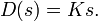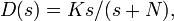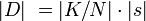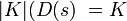# FAQ: How does a pole at high frequency prevent PID amplification of high frequency noise?We see that as gets large, so does <mat>|D|[/itex]. To offset this effect, what we need is a means to limit high frequency gain, i.e., to bound the value of. Let us consider a modified version of D(s) asSo how does this help? At low frequencies (small s), we see that(because N is chosen large). Therefore,. Thus at low frequencies, the transfer function behaves just like the plain differentiatior (Ks) - our old transfer function. At high frequencies (s large), we see that s swamps out N, and hence. The high frequency gain is therefore limited by.